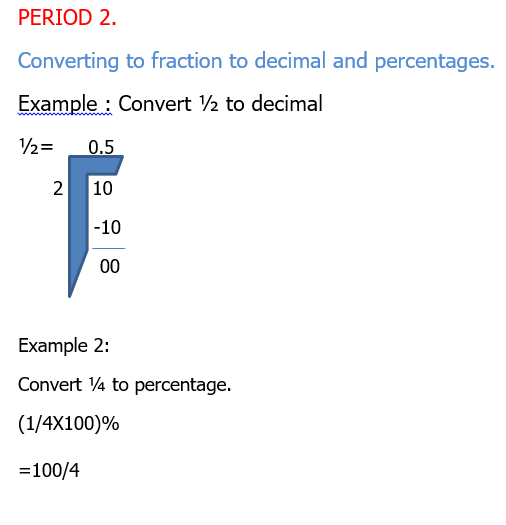### Fraction.2

Mathematics for JSS1 First Term

WEEK 7.

Fraction.

Performance Objectives.

Students should be able to

1. Arrange a given fraction either in ascending or descending order.
2. Convert fractions to decimal.
3. Convert decimal to fraction.
4. Convert fractions to percentages.
5. Convert percentages to fraction.

Content.

Example 1: Which is larger 2/7 and 6/7.

LCM of 7 is 7.

2/7x7=2

6/7x7=6

Therefore 6/7 is greater than 2/7.

Example 2: Arrange in ascending order ¼,2/5,7/8,1/2.

LCM of 4,5,8,2 is 40.

1/4x40=10.

2/5x40=16

7/8x40=35

1/2x40=20.

Ascending order(from the smallest to biggest) ¼,2/5,1/2,7/8.View More